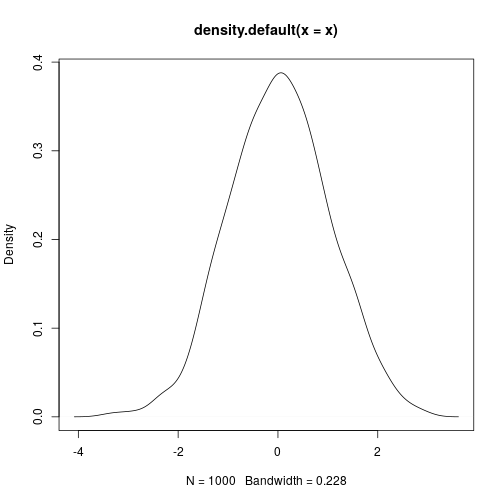# Literate programming

## Overview

Teaching: 30 min
Exercises: 10 min
Questions
• What tools are available to easily disseminate our work?

• How can we make our work more reproducible?

Objectives
• Learn the basics of RMarkdown and knitr

• Learn to integrate R code with text and images.

## 2. Literate programming

• make work more pleasant for yourself? (less tedium, less manual, less …)
• reduce friction for collaboration?
• reduce friction for communication?
• make your work navigable, interpretable, and repeatable by others?

Process, packaging, and presentation are often the weak links in the chain.## Markdown

### What is Markdown?

• Markdown is a particular type of markup language. Markup languages are designed to produce documents from plain text.
• You may be familiar with LaTeX, another (though less human friendly) text markup language.
• Tools render markdown to different formats (for example, HTML/pdf/Word).
• The main tool for rendering Markdown is pandoc.

Adapted from Carson Sievert’s markdown slides

### Markdown enables fast publication to the web

• Markdown Easy to write and read in an editor.
• HTML Easy to publish and read on web.

### Markdown versus HTML code

This is a Markdown document.

It's easy to do *italics* or __make things bold__.

> All models are wrong, but some are useful. An approximate answer to the right problem is worth a good deal more than an exact answer to an approximate problem.

Code block below. Just affects formatting here.


x <- 3 * 4


I can haz equations. Inline equations, such as the average is computed as $\frac{1}{n} \sum_{i=1}^{n} x_{i}$. Or display equations like this:

$$\begin{equation*} |x|= \begin{cases} x & \text{if x\ge 0,} \\\\ -x &\text{if x\lt 0.} \end{cases} \end{equation*}$$<!DOCTYPE html>
<html>
<meta http-equiv="Content-Type" content="text/html; charset=utf-8"/>
<title>Title</title>
<!-- MathJax scripts -->
<script type="text/javascript" src="..."></script>
<style type="text/css">
body {
font-family: Helvetica, arial, sans-serif;
font-size: 14px;
...
</style>
<body>
<p>This is a Markdown document.</p>

<p>It's easy to do <em>italics</em> or <strong>make things bold</strong>.</p>

<blockquote><p>All models are wrong, but some are...</p></blockquote>

<p>Code block below. Just affects formatting here.</p>

<pre><code>x <- 3 * 4</code></pre>### Markdown versus rendered HTML


This is a Markdown document.

It's easy to do *italics* or __make things bold__.

> All models are wrong, but some are useful. An approximate answer to the right problem is worth a good deal more than an exact answer to an approximate problem.

Code block below. Just affects formatting here.

r
x <- 3 * 4


I can haz equations. Inline equations, such as the average is computed as $\frac{1}{n} \sum_{i=1}^{n} x_{i}$. Or display equations like this:

$$\begin{equation*} |x|= \begin{cases} x & \text{if x\ge 0,} \\ -x &\text{if x\lt 0.} \end{cases} \end{equation*}$$This is a Markdown document.

It's easy to do italics or make things bold.

All models are wrong, but some are useful. An approximate answer to the right problem is worth a good deal more than an exact answer to an approximate problem.

Code block below. Just affects formatting here.

x <- 3 * 4

I can haz equations. Inline equations, such as the average is computed as $$\frac{1}{n} \sum_{i=1}^{n} x_{i}$$.

Or display equations like this:

$\begin{equation*} |x|= \begin{cases} x & \text{if x\ge 0,} \ -x & \text{if x\lt 0.} \end{cases} \end{equation*}$## Markdown can be rendered to multiple formats

• pandoc is a Swiss-army knife tool for conversion## RMarkdown

RMarkdown is rendered to Markdown. The strength of RMarkdown, is that with an easy syntax, it is possible to mix ideas, code, and generated results seamlessly.

### Input

This is an R Markdown document.

{r sec_3}
x <- rnorm(1000)


knitr offers a lot of control over representing different
types of output. We can also have inline R expressions
computed on the fly.

The mean $\bar{x} = \frac{1}{n} \sum_{i=1}^{n} x_{i}$ of the
r length(x) random variates we generated is
r round(mean(x), 3).

This figure is computed on-the-fly as well. No more
copy-paste, including for figures:

{r sec_4}
plot(density(x))



### Output

This is an R Markdown document.

x <- rnorm(1000)

##  -3.2538882  0.6478847 -2.3089858 -1.8085141  0.5498946  1.4029523


knitr offers a lot of control over representing different types of output. We can also have inline R expressions computed on the fly.

The mean $$\bar{x} = \frac{1}{n} \sum_{i=1}^{n} x_{i}$$ of the 1000 random variates we generated is 0.036.

This figure is computed on-the-fly as well. No more copy-paste, including for figures:

plot(density(x))## Rendering can be automated is thus repeatableFrom within R:

rmarkdown::render("filename.Rmd")

From the command line:

\$ Rscript -e "rmarkdown::render('filename.Rmd')"

## Summary

• RMarkdown enables ideas and questions, the code that implements them, and the results generated by the implementation, to all stay together.
• RMarkdown toolchain allows automated, repeatable rendering
• for publishing to the web and viewing through a browser
• and (through LaTeX) to obtain a submittable manuscript (in PDF or Word).
• knitr is not limited to executing R code. See the book Dynamic documents with R and knitr by Yihui Xie, part of the CRC Press / Chapman & Hall R Series (2013). ISBN

## Key Points

• RMarkdown and knitr` are powerful tools.

• Output can be rendered in many formats.

• You don’t need to know HTML!